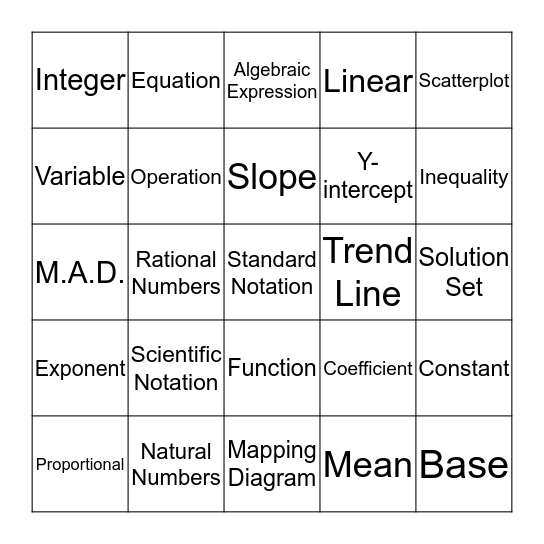# Math Success Vocabulary BINGO!!!!!!This bingo card has a free space and 24 words: Scientific Notation, Natural Numbers, Slope, Proportional, Scatterplot, Integer, Variable, Equation, Trend Line, Function, Y-intercept, M.A.D., Coefficient, Constant, Operation, Mean, Solution Set, Algebraic Expression, Inequality, Rational Numbers, Base, Exponent, Linear and Mapping Diagram.

## Play Online